NCERT Questions

Class 10
Chapter 12 Class 10 - Electricity

## Compare the power used in the 2 Ω resistor in each of the following circuits: (i) a 6 V battery in series with 1 Ω and 2 Ω resistors, and (ii) a 4 V battery in parallel with 12 Ω and 2 Ω resistors.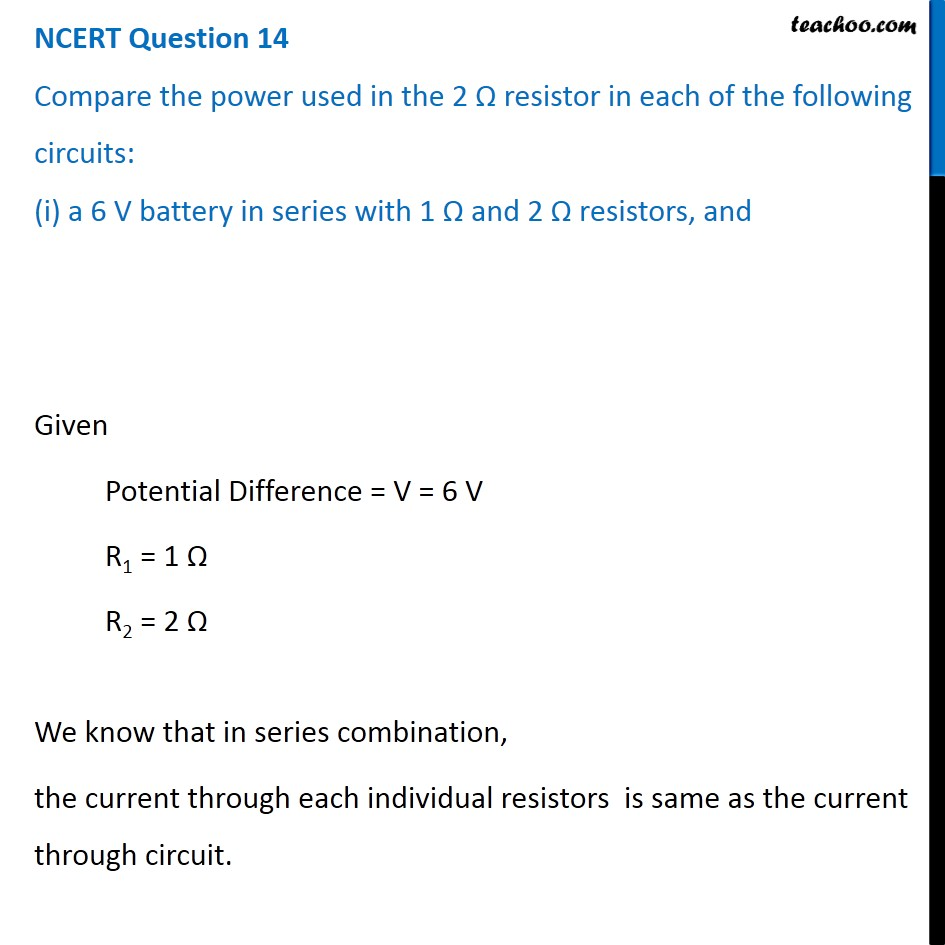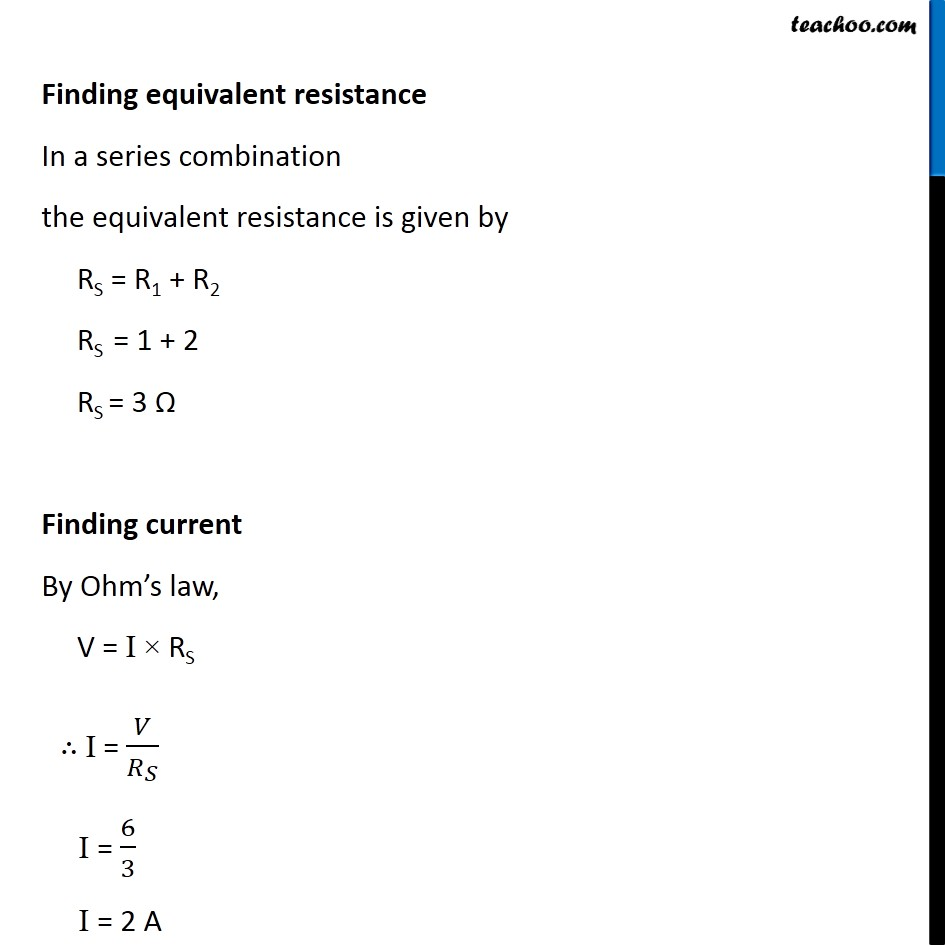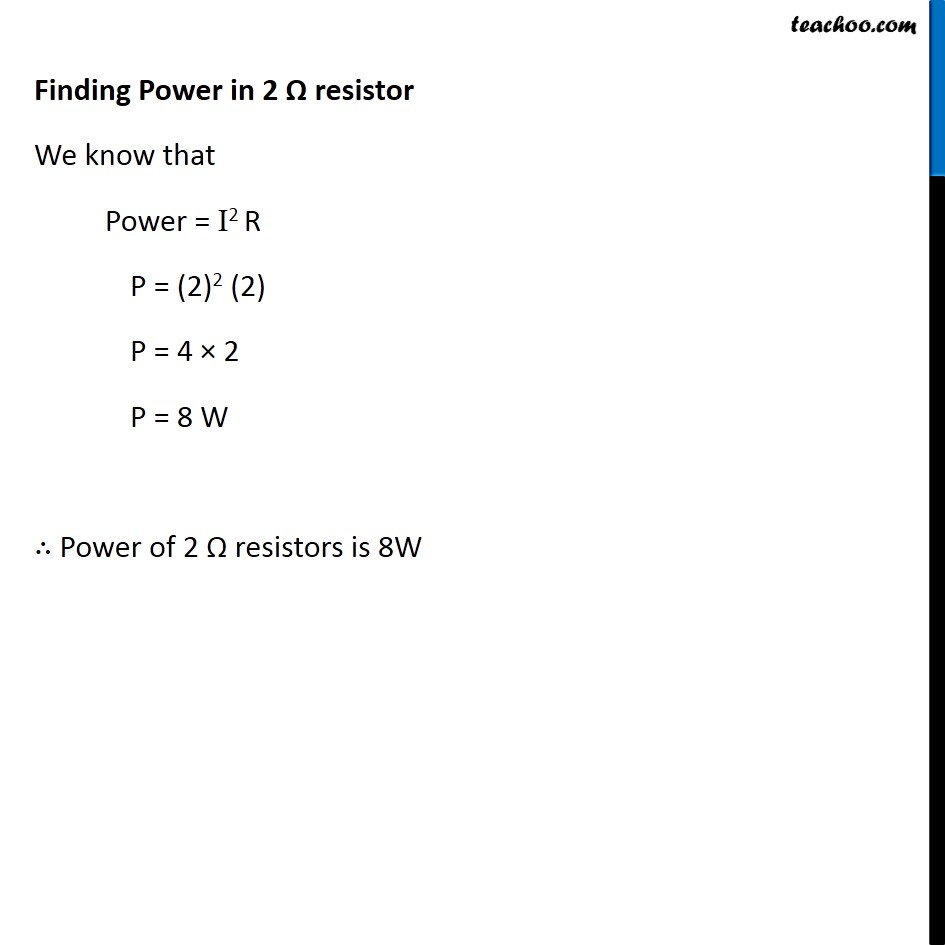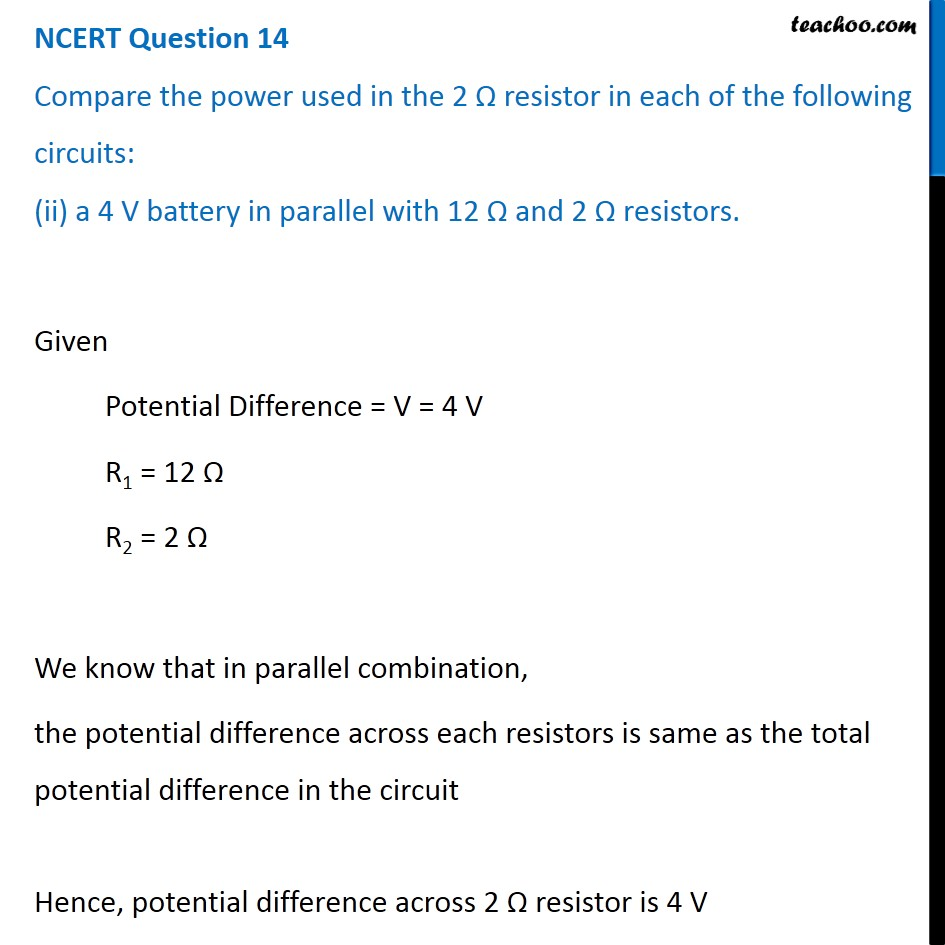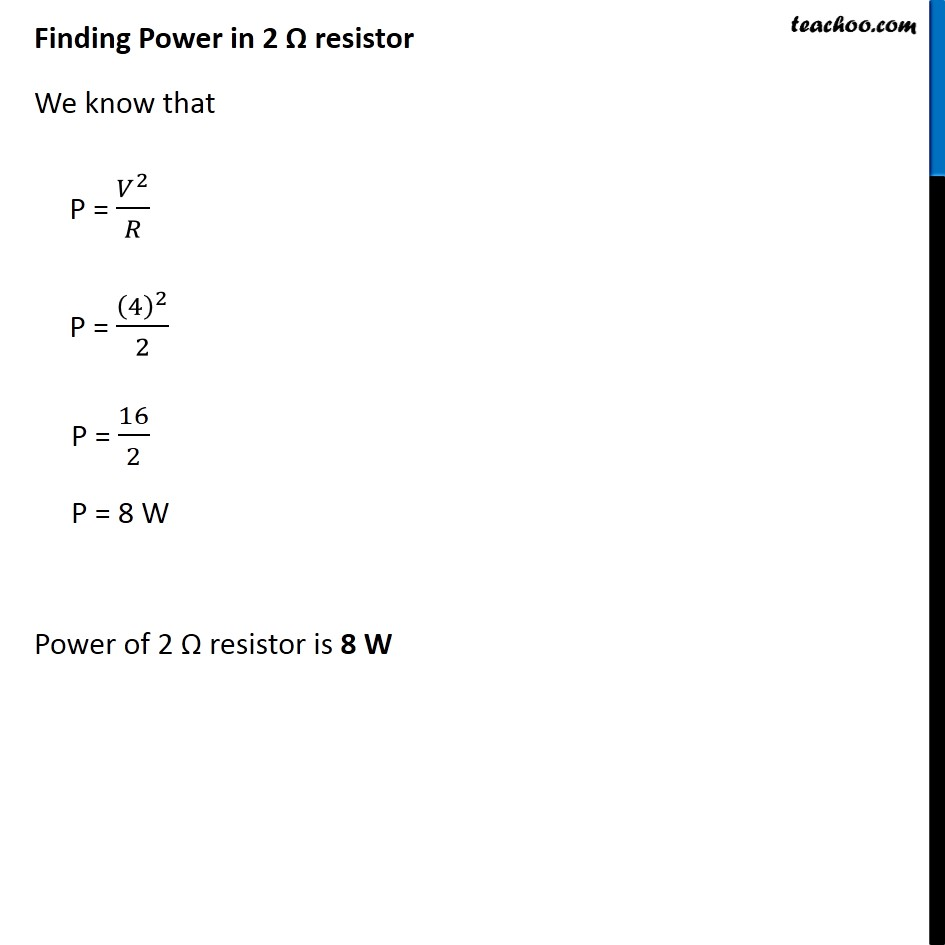Learn in your speed, with individual attention - Teachoo Maths 1-on-1 Class

### Transcript

NCERT Question 14 Compare the power used in the 2 Ω resistor in each of the following circuits: (i) a 6 V battery in series with 1 Ω and 2 Ω resistors, and Given Potential Difference = V = 6 V R1 = 1 Ω R2 = 2 Ω We know that in series combination, the current through each individual resistors is same as the current through circuit. Finding equivalent resistance In a series combination the equivalent resistance is given by RS = R1 + R2 RS = 1 + 2 RS = 3 Ω Finding current By Ohm’s law, V = I × RS ∴ I = 𝑉/𝑅_𝑆 I = 6/3 I = 2 A Finding Power in 2 Ω resistor We know that Power = I2 R P = (2)2 (2) P = 4 × 2 P = 8 W ∴ Power of 2 Ω resistors is 8W NCERT Question 14 Compare the power used in the 2 Ω resistor in each of the following circuits: (ii) a 4 V battery in parallel with 12 Ω and 2 Ω resistors. Given Potential Difference = V = 4 V R1 = 12 Ω R2 = 2 Ω We know that in parallel combination, the potential difference across each resistors is same as the total potential difference in the circuit Hence, potential difference across 2 Ω resistor is 4 V Finding Power in 2 Ω resistor We know that P = 𝑉^2/𝑅 P = (4)^2/2 P = 16/2 P = 8 W Power of 2 Ω resistor is 8 W